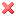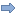# Stat-2160-145 homework 6 business statistics

## Quiz Submissions – Homework 6

Question 1
You are curious about the average number of yards Matthew Stafford throws for each game for the Detroit Lions. You randomly select 29 games and see that the average yards per game is 276.9 with a standard deviation of 38.37 yards. You want to create a 95% confidence interval for the true average number of yards per game he throws. What is the margin of error for this estimate?

1) 12.1208

2) 14.5952

3) 1.6463

4) 14.5725

5) 7.1251

Question 2

The owner of a local phone store wanted to determine how much customers are willing to spend on the purchase of a new phone. In a random sample of 15 phones purchased that day, the sample mean was \$429.888 and the standard deviation was \$21.1186. Calculate a 99% confidence interval to estimate the average price customers are willing to pay per phone.

1) ( 426.911 , 432.865 )

2) ( 413.819 , 445.957 )

3) ( 424.435 , 435.341 )

4) ( 413.656 , 446.12 )

5) ( -413.656 , 446.12 )

Question 3

In a group of 18 young professionals studied, it was found that they purchased on average \$219.838 per month eating meals prepared outside the home, with a standard deviation of \$37.6396. What is the 95% confidence interval of the true amount of money young professionals spend monthly on meals prepared outside the home?

1) ( 210.966 , 228.71 )

2) ( 201.12 , 238.556 )

3) ( -201.12 , 238.556 )

4) ( 201.198 , 238.478 )

5) ( 217.728 , 221.948 )

Question 4

The owner of a local golf course wanted to determine the average age (in years) of the golfers that played on the course. In a random sample of 30 golfers that visited his course, the sample mean was 46.3 years old and the standard deviation was 6.81 years. Using this information, the owner calculated the confidence interval of (42.9, 49.7) with a confidence level of 99% for the average age. Which of the following is an appropriate interpretation of this confidence interval?

1) We are 99% confident that the average age of all golfers that play on the golf course is between 42.9 and 49.7 years old.

2) We are 99% confident that the average age of the golfers surveyed is between 42.9 and 49.7 years old.

3) We are certain that 99% of the average ages of all golfers will be between 42.9 and 49.7 years old.

4) We are 99% confident that the proportion of the ages of all golfers is between 42.9 and 49.7 years old.

5) We cannot determine the proper interpretation of this interval.

Question 5

A local pizza place claims that they average a delivery time of 7.75 minutes. To test this claim, you order 14 pizzas over the next month at random times on random days of the week. You calculate that the average delivery time is 8.68 minutes with a standard deviation of 1.382 minutes. You create a 90% confidence interval of (8.03, 9.33). Of those listed below, what is the best conclusion you can make?

1) You are 90% confident that the average delivery time is greater than 7.75 minutes.

2) You are 90% confident that the average delivery time is less than 7.75 minutes.

3) The percentage of pizzas that arrive around 7.75 minutes is 90%.

4) The average delivery time does not significantly differ from 7.75 minutes.

5) We cannot determine the proper interpretation based on the information given.

Question 6

As an avid golfer, you want to estimate your average score for 18 holes of golf. Suppose you know that the standard deviation of your score is 24.621 strokes and you want to find a sample mean that is within 6.271 strokes of your true average for all rounds of golf with 99% confidence. How many rounds would you need to play to determine this?1) 1022) 103

3) 108

4) 113

5) We do not have enough information to answer this question since we were not given the sample mean.

Question 7

In a consumer research study, several Meijer and Walmart stores were surveyed at random and the average basket price was recorded for each. It was found that the average basket price for 35 Meijer stores was \$51.376 with a standard deviation of \$12.892. Similarly, 49 Walmart stores had an average basket price of \$51.138 with a standard deviation of \$14.332. If a 95% confidence interval for the difference between the true average basket prices of Meijer versus Walmart is calculated, what is the margin of error? You can assume that the standard deviations of the two populations are statistically similar.

1) 5.966

2) 5.064

3) 1.98931856

4) 6.055

5) 3.04377454

Question 8

It is believed that using a solid state drive (SSD) in a computer results in faster boot times when compared to a computer with a traditional hard disk (HDD). You sample 15 computers with an HDD and note a sample average of 20.656 seconds with a standard deviation of 4.1958 seconds. A sample of 10 computers with an SSD show an average of 14.939 seconds with a standard deviation of 2.806 seconds. Construct a 99% confidence interval for the difference between the true average boot times of the two types of hard drives. Assume the difference will represent (HDD-SSD) and that the population standard deviations are statistically the same.

1) (1.46, 9.974)

2) (-10.096, 21.53)

3) (1.811, 9.623)

4) (4.201, 7.233)

5) We only have the sample means, we need to know the population means in order to calculate a confidence interval.

Question 9

A pharmaceutical company is testing a new drug to increase memorization ability. It takes a sample of individuals and splits them randomly into two groups. One group is administered the drug, and the other is given a placebo. After the drug regimen is completed, all members of the study are given a test for memorization ability with higher scores representing a better ability to memorize. You are presented a 90% confidence interval for the difference in population mean scores (with drug – without drug) of (-17.5, -4.5). What can you conclude from this interval?

1) We are 90% confident that the average memorization ability of those on the drug is higher than those who are not on the drug.

2) There is no significant difference between the average memorization abilities for those on the drug compared to those not on the drug.

3) We are 90% confident that the average memorization ability of those not on the drug is higher than those who are on the drug.

4) We are 90% confident that the difference between the two sample means falls within the interval.

5) We do not have enough information to make a conclusion.

Question 10

The owner of a local golf course wants to estimate the difference between the average ages of males and females that play on the golf course. He randomly samples 30 men and 22 women that play on his course. He finds the average age of the men to be 34.666 with a standard deviation of 8.002. The average age of the women was 36.618 with a standard deviation of 7.828. He uses this information to calculate a 95% confidence interval for the difference in means, (-6.424, 2.52). The best interpretation of this interval is which of the following statements?

1) We are 95% confident that the difference between the average age of all men and all women who play golf at the course is between -6.424 and 2.52

2) We do not know the population means so we do not have enough information to make an interpretation.

3) We are certain that the difference between the average age of all men and all women is between -6.424 and 2.52.

4) We are 95% confident that the difference between the average age of the men and women surveyed is between -6.424 and 2.52

5) We are 95% sure that the average age difference between all males and females is between -6.424 and 2.52.

Question 11

Researchers at the corporate office of an airline wonder if there is a significant difference between the cost of a flight on Priceline.com vs. the airline’s own website. A random sample of 29 flights were tracked on Priceline and the airline’s website and the mean difference in price (Priceline – Airline Site) was \$-55.175 with a standard deviation of \$12.403. If you wanted to create a 95% confidence interval for the true average difference in costs between the vendors, what is the margin of error?

1) 4.7178

2) 2.3032

3) 4.7105

4) 3.9134

5) 4.5142

Question 12

A restaurant wants to test a new in-store marketing scheme in a small number of stores before rolling it out nationwide. The new ad promotes a premium drink that they want to increase the sales of. 10 locations are chosen at random and the number of drinks sold are recorded for 2 months before the new ad campaign and 2 months after. The average difference in the sales quantity (after – before) is -108.801 with a standard deviation of 48.8204. Calculate a 95% confidence interval to estimate the true average difference in nationwide sales quantity before the ad campaign and after.

1) (-143.725, -73.877)

2) (143.725, -73.877)

3) (-124.2394, -93.3626)

4) (-111.0632, -106.5388)

5) (-143.1998, -74.4022)

Question 13

Researchers in the corporate office of an airline wonder if there is a significant difference between the cost of a flight on Priceline.com vs. the airline’s own website. A random sample of 17 flights were tracked on Priceline and the airline’s website and the 90% confidence interval for the mean difference in price (Priceline – Airline Site) was (-183.62, -91.37). Which of the following is the appropriate conclusion?

1) We are 90% confident that the average difference in price is positive, with the higher price coming from Priceline.

2) We are 90% confident that the average difference in price is negative, with the higher price coming from the Airline sites

3) There is no significant difference between the average price for the booking options

4) We are 90% confident that the average difference in price is positive, with the higher price coming from the Airline sites

5) We are 90% confident that the average difference in price is negative, with the higher price coming from Priceline.

Question 14

Automobile manufacturers are interested in the difference in reaction times for drivers reacting to traditional incandescent lights and to LED lights. A sample of 22 drivers are told to press a button as soon as they see a light flash in front of them and the reaction time was measured in milliseconds. Each driver was shown each type of light. The average difference in reaction times (traditional – LED) is 0.1 ms with a standard deviation of 5.27 ms. A 95% confidence interval for the average difference between the two reaction times was (-2.24, 2.44). Which of the following is the best interpretation?

1) We are certain the average difference in reaction times between the two light types for all drivers is between -2.24 and 2.44.

2) We are 95% confident that the average difference in the reaction times of the drivers sampled is between -2.24 and 2.44.

3) We are 95% confident that the average difference in reaction time between the two light types for all drivers is between -2.24 and 2.44.

4) We are 95% confident that the difference between the average reaction time for LED lights and the average reaction time for traditional lights is between -2.24 and 2.44.

5) The proportion of all drivers that had a difference in reaction times between the two lights is 95%.

Attempt Score:14 / 14 – A

Overall Grade (average of all attempts):14 / 14

"We Offer Paper Writing Services on all Disciplines, Make an Order Now and we will be Glad to Help"